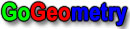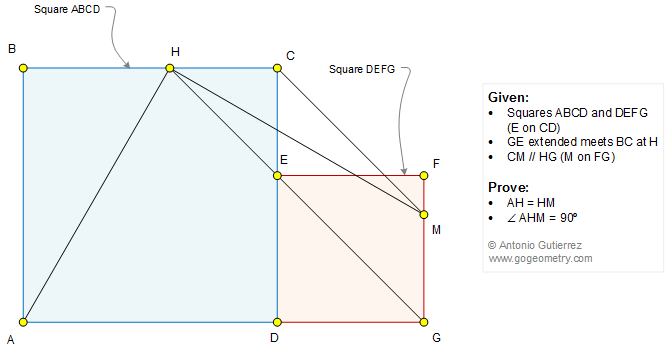#Problem 1329: Two Squares Side by Side, Parallel, Perpendicular, 90 Degrees, Congruence.

 < PREVIOUS PROBLEM  |  NEXT PROBLEM > The figure below shows the squares ABCD and DEFG (E on CD). GE extended meets BC at H. CM is parallel to HG (M on FG). Prove that (1) AH = HM; (2) angle AHM = 90 degrees.See also: Art of problem 1312

 Home | Geometry | Problems | All Problems | Open Problems | Visual Index | Ten problems: 1321-1330 | Triangle | Square | Parallel lines | Perpendicular line | Angle | Congruence | View or Post a solution | by Antonio Gutierrez